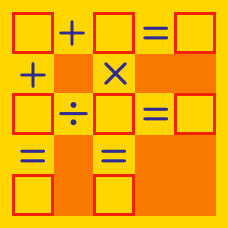Logic

# Arithmetic Puzzles: Level 4 Challenges

Given a positive integer $n$, let $p(n)$ be the product of the non-zero digits of $n$. (If $n$ has one digit, then $p(n)$ is equal to that digit.) Let

$S = p(1) + p(2) + \cdots + p(999).$

What is the largest prime factor of $S$?

$12345678910111213141516 \ldots$

The number above shows a concatenating of the natural numbers in ascending order. What is the $28383^\text{rd}$ digit from the left (1 being the first) of the number above?

Three candles which can burn, 60 minutes, 80 minutes and 100 minutes respectively are lit at different times. All the candles are burning simultaneously for 30 minutes, and there is a total of 40 minutes in which exactly one is burning. For how many minutes are exactly two candles burning?

Krishna always reads some (at least 2) pages of "Harry Potter" before going to school. One good day, Agnishom asked him - "Krishna, what is the sum of all the page numbers you read today?"

Krishna replied "It is either 512 or 412."

What is it?

For any positive integer $k$ let $f_1(k)$ denote the sum of the squares of the digits of $k$ (when written in decimal), and for $n \ge 2$ define $f_n(k)$ iteratively by $f_n(k)=f_1(f_{n-1}(k))$.

Find $f_{2017}(2016)$.

×